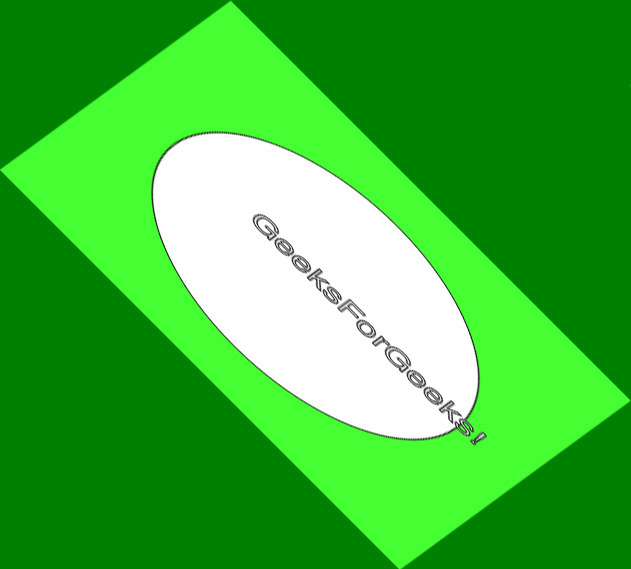Related Articles
Wand shear() function – Python
• Last Updated : 04 May, 2020

The shear() function is an inbuilt function in the Python Wand ImageMagick library which is used to slide one edge of an image along the X or Y axis to create a parallelogram. The X-direction shear slides an edge along the X-axis, while a Y direction shear slides an edge along the Y-axis. The shear angle is used to set shear of the image.

Syntax:

`shear(background, x, y)`

Parameters: This function accepts four parameters as mentioned above and defined below:

• background: This parameter stores the background color.
• x: This parameter is used to set degrees to x-shear of the image.
• y: This parameter is used to set degrees to y-shear of the image.

Return Value: This function returns the Wand ImageMagick object.

Original Image:Example 1:

 `# Import library from Image ``from` `wand.image ``import` `Image`` ` `# Import the image``with Image(filename ``=``'../geeksforgeeks.png'``) as image:``    ``# Clone the image in order to process``    ``with image.clone() as shear:``        ``# Invoke shear function ``        ``shear.shear(``'Red'``, ``20``, ``30``)``        ``# Save the image``        ``shear.save(filename ``=``'shear1.jpg'``)`

Output:Example 2:

 `# Import libraries from the wand  ``from` `wand.image ``import` `Image``from` `wand.drawing ``import` `Drawing``from` `wand.color ``import` `Color`` ` `with Drawing() as draw:``    ``# Set Stroke color the circle to black``    ``draw.stroke_color ``=` `Color(``'black'``)``    ``# Set Width of the circlw to 2 ``    ``draw.stroke_width ``=` `1``    ``# Set the fill color to 'White (# FFFFFF)'``    ``draw.fill_color ``=` `Color(``'white'``)`` ` `    ``# Invoke Circle function with center at 50, 50 and radius 25``    ``draw.circle((``200``, ``200``), ``# Center point``                ``(``100``, ``100``)) ``# Perimeter point``    ``# Set the font style``    ``draw.font ``=` `'../Helvetica.ttf'``    ``# Set the font size ``    ``draw.font_size ``=` `30``     ` `    ``with Image(width ``=` `400``, height ``=` `400``, background ``=` `Color(``'# 45ff33'``)) as pic:``        ``# Set the text and its location``        ``draw.text(``int``(pic.width ``/` `3``), ``int``(pic.height ``/` `2``), ``'GeeksForGeeks !'``)``        ``# Draw the picture``        ``draw(pic)``        ``# Invoke shear function method``        ``pic.shear(``'Green'``, ``30``, ``45``)``        ``# Save the image ``        ``pic.save(filename ``=``'shear2.jpg'``)`

Output:Attention geek! Strengthen your foundations with the Python Programming Foundation Course and learn the basics.

To begin with, your interview preparations Enhance your Data Structures concepts with the Python DS Course. And to begin with your Machine Learning Journey, join the Machine Learning – Basic Level Course

My Personal Notes arrow_drop_up